# DAV Class 8 Maths Chapter 4 Worksheet 2 Solutions

The DAV Class 8 Maths Book Solutions and DAV Class 8 Maths Chapter 4 Worksheet 2 Solutions of Direct and Inverse Variation offer comprehensive answers to textbook questions.

## DAV Class 8 Maths Ch 4 WS 2 Solutions

Question 1.
In the following tables, a and b vary inversely. Fill in the missing values.

(i)Solution:
Let us put m and n in the blanks.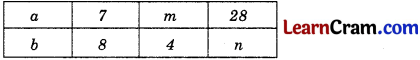As there is an inverse variation,
x1y2 = x2y1
⇒ 7 × 8 = m × 4
⇒ m = $$\frac{7 \times 8}{4}$$
⇒ m = 14
similarly, x1y1 = x2y2
⇒ 7 × 8 = 28 × n
⇒ n = $$\frac{7 \times 8}{28}$$
⇒ n = 2.
Hence, the missing values are 14 and 2.(ii)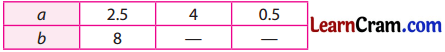Solution:
Let us put p and q in the blanks.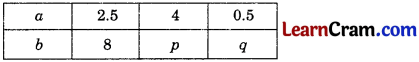x1y2 = x2y1
⇒ 2.5 × 8 = 4 × p
⇒ p = $$\frac{2.5 \times 8}{4}$$
⇒ p = 5
Similarly, x1y1 = x2y2
⇒ 2.5 × 8 = 0.5 × q
⇒ q = $$\frac{2.5 \times 8}{0.5}$$
⇒ q = 40.
Hence, the missing values are 5 and 40.

(iii)Solution:
Let us put s and t in the blanks.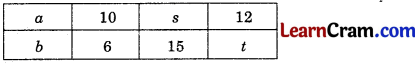As there is an inverse variation,
∴ x1y2 = x2y1
⇒ 10 × 6 = s × 15
⇒ s = $$\frac{10 \times 6}{15}$$
⇒ s = 4.
Similarly, x1y1 = x2y2
⇒ 10 × 6 = 12 × t
⇒ t = $$\frac{10 \times 6}{12}$$
⇒ t = 5
Hence, the missing values are 4 and 5.Question 2.
The science teacher asked the students of class-VIII to make a project report on pollution. When 10 students work on it, the work gets finished in 3 days. How many students are required so that the work finishes in 2 days?
Solution:
Let the number of students required be x.As there is an inverse variation,
x1y1 = x2y2
⇒ 10 × 3 = x × 2
⇒ x = $$\frac{10 \times 3}{2}$$
⇒ x = 15
Hence, the number of students required is 15.

Question 3.
Running at an average speed of 40 km/hr, a bus completes a journey in 4$$\frac{1}{2}$$ hours. How much time will the return journey take if the speed is increased to 45 km/hr?
Solution:
Let the time required be x hours.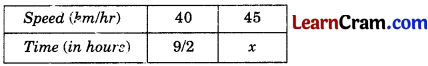As there is an inverse variation,
x1y1 = x2y2
⇒ 40 × $$\frac{9}{2}$$ = 45 × x
⇒ x = $$\frac{40 \times \frac{9}{2}}{45}$$
⇒ x = 4
Hence, the time required is 4 hours.

Question 4.
Disha cycles to her school at an average speed of 12 km/hr. It takes her 20 minutes to reach the school. If she wants to reach her school in 15 minutes, what should be her average speed?
Solution:
Let the required speed be x km/hr.As there is an inverse variation,
x1y1 = x2y2
⇒ 12 × 20 = x × 15
⇒ x = $$\frac{12 \times 20}{15}$$
⇒ x = 16
Hence, the required speed is 16 km/hr.Question 5.
If 15 men can repair a road in 24 days, then how long will it take 9 men to repair the same road?
Solution:
Let the required time be x days.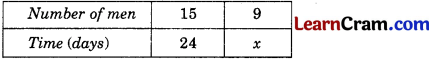As there is an inverse variation,
x1y1 = x2y2
⇒ 15 × 24 = 9 × x
⇒ x = $$\frac{15 \times 24}{9}$$
⇒ x = 40
Hence, the number of days required is 40.

Question 6.
If 30 goats can graze a field in 15 days, then how many goats will graze the same field in 10 days?
Solution:
Let the number of goats be x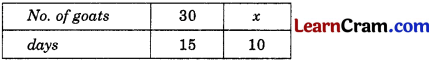As there is an inverse variation,
x1y1 = x2y2
⇒ 30 × 15 = x × 10
⇒ x = $$\frac{30 \times 15}{10}$$
⇒ x = 45
Hence the required number of goats is 45.

Question 7.
A contractor with a work force of 420 men can complete a work of construction of a building in 9 months. Due to request by the owners, he was asked to complete the job in 7 months. How many extra men, he must employ to complete the job?
Solution:
Let number of extra men required be x.As there is an inverse variation,
x1y1 = x2y2
⇒ 420 × 9 = x × 7
⇒ x = $$\frac{420 \times 9}{7}$$
⇒ x = 540
Extra men required = 540 – 420 = 120
Hence the extra men required = 120.Question 8.
Uday can finish a book in 25 days, if he reads 18 pages every day. How many days will he take to finish it, if he reads 15 pages every day?
Solution:
Let the number of days required be x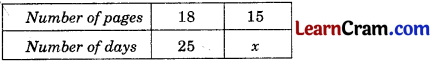As there is an inverse variation,
x1y1 = x2y2
⇒ 18 × 25 = 15 × x
⇒ x = $$\frac{40 \times 125}{100}$$
⇒ x = 30
Hence, the number of days required = 30 days.

Question 9.
A {shopkeeper has enough money to buy 40 books, each costing ₹ 125. How many books he can buy if he gets a discount of ₹ 25 on each book?
Solution:
Let the number of books required be x.Cost after discount = 125 – 25 = ₹ 100
As there is an inverse variation,
x1y1 = x2y2
⇒ 40 × 125 = x × 100
⇒ x = $$\frac{40 \times 125}{100}$$
⇒ x = 50
Hence, the number of books = 50.

Question 10.
Six pumps working together empty a tank in 28 minutes. How long will it take to empty the tank if 4 such pumps are working together?
Solution:
Let the required time be x minutes.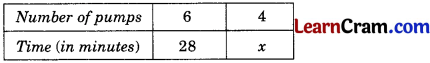As there is an inverse variation.
x1y1 = x2y2
⇒ 6 × 28 = 4 × x
⇒ x = $$\frac{6 \times 28}{4}$$
⇒ x = 42
Hence, the time required is 42 minutes.Question 11.
A train moving at a speed of 75 km/hr covers a certain distance in 4.8 hours. What should be the speed of the train to cover the same distance in 3 hours?
Solution:
Let the required speed be x km/hr.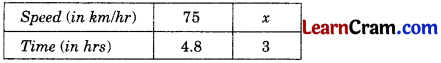As there is an inverse variation,
x1y1 = x2y2
⇒ 75 × 4.8 = x × 3
⇒ x = $$\frac{75 \times 4.8}{3}$$
⇒ x = 120
Hence, the speed need is 120 km/hr.

Question 12.
A garrison of 120 men has provision for 30 days. At the end of 5 days, 5 more men joined them. How many days can they sustain on the remaining provision?
Solution:
Let the number of days required be x.
Now the number of men becomes 120 + 5 = 125
Number of days left = 30 – 5 = 25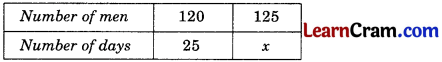As there is an inverse variation,
x1y1 = x2y2
⇒ 120 × 25 = 125 × x
⇒ x = $$\frac{120 \times 25}{125}$$
⇒ x = 24
Hence, the provision will last for 24 days.### DAV Class 8 Maths Chapter 4 Worksheet 2 Notes

Inverse Variation

Example 1.
6 Pipes are required to fill a tank in 1 hour 20 minutes. How long will it take if only 5 pipes of the sanie type are used to fill the same tank?
Solution:
Let the required time be x hrs.

 Number. of pipes 6 5 Time (in hours) 4/3 x

[∵ 1 hour 20 minutes = 1 + $$\frac{1}{3}$$ = $$\frac{4}{3}$$ hours]

As there is inverse variation,
x1y1 = x2y2
⇒ 6 × $$\frac{4}{3}$$ = 5 × x
⇒ x = $$\frac{6 \times \frac{4}{3}}{5}$$
⇒ x = $$\frac{8}{5}$$ = 1 hour 36 minutes.
Hence, the required time is 1 hour 36 minutes.

Example 2.
If 15 workers can build a wall in 48 hours, how many workers will be required to do the same work in 30 hours?
Solution:
Let the number of workers required be x.

 Number of Workers 15 x Time (in hrs.) 48 30

As there is inverse variation,
x1y1 = x2y2
⇒ 15 × 48 = x × 30
⇒ x = $$\frac{15 \times 48}{30}$$
⇒ x = 24
Hence the number of workers required is 24.Example 3.
A car takes 2 hours to reach a destination by travelling at the speed of 60 km/hr. How long will it take when the car travels at the speed of 80 km/hr?
Solution:
Let the time required be x hrs.

 Time (in hours) 2 x Speed (km/hr) 60 80

As there is an inverse variation,
x1y1 = x2y2
⇒ 2 × 60 = x × 80
⇒ x = $$\frac{2 \times 60}{80}$$
⇒ x = $$\frac{3}{2}$$ hrs
= 1 hour 30 minutes.
Hence, the required time is 1 hour 30 minutes.

Example 4.
If 52 men can do a piece of work in 35 days. In how many days will 28 men complete the same work?
Solution:
Let the number of days required be x.

 Number of men 52 28 Number of days 35 x

As there is an inverse variation,
x1y1 = x2y2
⇒ 52 × 35 = 28 × x
⇒ x = $$\frac{52 \times 35}{28}$$
⇒ x = 65
Hence, the number of days required is 65.